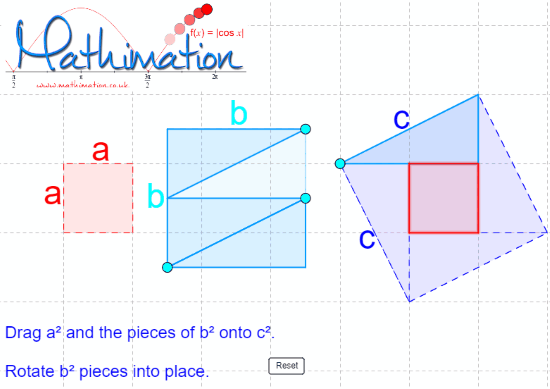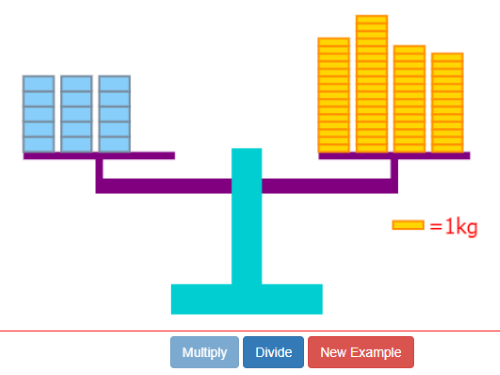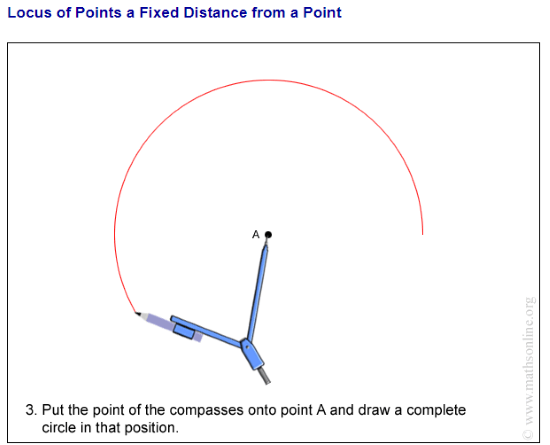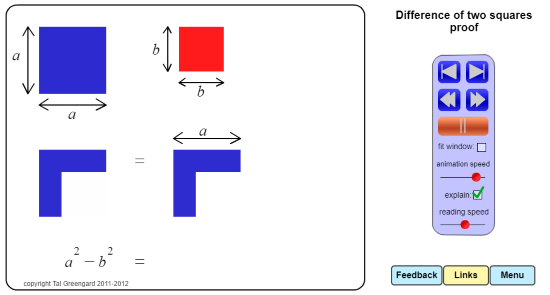Editor Ratings:
User Ratings:
[Total: 2 Average: 1]

Here is the list of 5 online Interactive Math lessons websites for free. Now you can make your Math subject more interesting to understand. The sites have different topics such as angles, triangles, Geometry, Algebra, Probability scale drawing animations, etc. Each title has sub-topics. You can read definitions, look for graphs, diagrams, etc.

The good thing about these sites is you can find good explanations of mathematics and visual concepts. You can practice questions. The sites have solutions as well. Sometimes, students find their textbook boring and hard to understand. That’s why I have covered these sites for better understanding. You can practice Math with animation and illustrations. Also, you can also use online calculators with steps and store each step of calculations.

## 1. MathigonMathigon is a free website where you can learn Interactive Math lessons. The site has amazing visuals, animations, and illustrations. You can use Math glossary as well.

You can browse courses related to Math subject. The Mathigon course library has topics such as Geometry, Algebra, Probability, etc. Each topic has sub-topics where you can read in details about the Math subject. It presents graph and diagrams in a way as someone is drawing it for you to teach. Not just this, you can also practice by answering the questions.

The interesting part about this site is that it makes your reading interesting with animations and illustrations. For example, if you open a topic Circle, then the site illustrates circles and diagrams. Besides that, the site provides videos which you can watch for a better understanding.

## 2. MathimationMathimation is another website for Interactive Math lessons. The site teaches you Mathematics with visualizations and animations.

The site has topics like Graphs, Circles, Numbers, Statistics, Transformations, Angles, Triangles, and Shapes. If you click on the topic, it shows different sub-topics which you can read one by one. You can read the definitions while looking at the graphs. You can zoom in/out the picture.

Also, the site teaches you to follow instructions to solve the sums. You can solve the diagrams online. As shown in the image above, you can drag the first two diagrams and drop into the third diagram to complete it. In this way, you can learn numbers, statistics, etc.

## 3. Animated MathematicsAs the name suggests, the site provides animated mathematics lessons. The site lets you understand Mathematics with visuals and animations. It has examples and questions for practice with complete solutions.

The site has lessons like basics, Algebra, Straight lines, Quadratics, Calculus, Math, and programming. When you open a topic, you will see sub-topics below the main topic. Then, you can choose the topic to read and practice with great visuals. Also, there are a lot of examples. If you don’t understand the example, you can move to another example and understand Math rather than memorizing it.

Besides that, the site has the latest topics on the homepage, you may go through that too. Every question has a solution with clear graphs.

## 4. Maths OnlineMaths Online is another great option for Interactive Math lessons. Using this website, you can understand the Math subject in an interesting way.

The site has different subjects like multiplication table, long division, recognizing shapes and many more. There is a section named Animation. You can find animations and great visuals. This particular category has different topics like angles, triangles, scale drawing animations. Each topic takes a few seconds to start the animations. And then, you can practice Math with good animations. Also, the site instructs you to make graph online.

## 5. SeeMath.ComSeeMath.Com is a free website for Interactive Math lessons. The site provides you animations, visuals, and other mathematical concepts.

When you visit the site, it shows categories on the top. You can look for numbers, algebra menu, featured. Also, you can use the search bar to find a specific topic. If you go to the number section, you will see topics related to the numbers only, e.g., whole numbers, negative numbers, fractions, etc. Similarly, the algebra part has covered topics like operations, substitutions, equations, etc.

Once you open a topic, it shows an animator player on the right. You can play that and on the left, the sum keeps on solving. You can go forward and backward. If you find the speed fast, you can set the animation speed.

### In brief

There are other websites where you can browse course or subjects related to Math subject. But using the above-mentioned sites, you can make your subject more interesting to understand. The sites provide you quick solutions with great visual concepts. Also, you can practice Math with illustrations.

 Editor Ratings: User Ratings:[Total: 2 Average: 1] Tags: mathematics